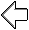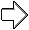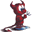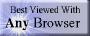music# Assembly Language

## summary

This web page examines floating point arithmetic instructions in assembly language. Specific examples of instructions from various processors are used to illustrate the general nature of assembly language.

## free computer programming text book project

If you like the idea of this project,Now building a For those with high speed connections, the very large single file summary is still on line.

### floating point arithmetic

For most processors, integer arithmetic is faster than floating point arithmetic. This can be reversed in special cases such digital signal processors.

On many processors, floating point arithmetic is in an optional unit or optional coprocessor rather than being included on the main processor. This allows the manufacturer to charge less for the business machines that don’t need floating point arithmetic.

The basic four floating point arithmetic operations are addition, subtraction, multiplication, and division. Some processors don’t include actual multiplication or division hardware, instead looking up the answer in a massive table of results embedded in the processor.

Compare instructions are used to examine one or more floating point numbers non-destructively. These are usually implemented by performing a subtraction in some shadow register or accumulator and then setting flags accordingly. Compare instructions can compare two floating point numbers, or can compare a single floating point number to zero.

• SUB Subtract; DEC VAX; signed subtraction of scalar quantities (32, 64, or 128 bit floating point) in general purpose registers or memory, available in two operand (first operand subtracted from second operand with result replacing second operand) and three operand (first operand subtracted from second operand with result placed in third operand) (SUBF2 subtract float 2 operand, SUBF3 subtract float 3 operand, SUBD2 subtract double float 2 operand, SUBD3 subtract double float 3 operand, SUBG2 subtract G float 2 operand, SUBG3 subtract G float 3 operand, SUBH2 subtract H float 2 operand, SUBH3 subtract H float 3 operand); clears or sets flags
• MUL Multiply; DEC VAX; signed multiplication of scalar quantities (32, 64, or 128 bit floating point) in general purpose registers or memory, available in two operand (first operand multiplied by second operand with result replacing second operand) and three operand (first operand multiplied by second operand with result placed in third operand) (MULF2 multiply float 2 operand, MULF3 multiply float 3 operand, MULD2 multiply double float 2 operand, MULD3 multiply double float 3 operand, MULG2 multiply G float 2 operand, MULG3 multiply G float 3 operand, MULH2 multiply H float 2 operand, MULH3 multiply H float 3 operand); clears or sets flags
• EMOD Extended Multiply and Integerize; DEC VAX; performs accurate range reduction of math function arguments, the floating point multiplier extension operand (second operand) is concatenated with the floating point multiplier (first operand) to gain eight additional low order fraction bits, the multiplicand operand (third operand) is multiplied by the extended multiplier operand, after multiplication the integer portion (fourth operand) is extracted and a 32 bit (EMODF) or 64 bit (EMODD) floating point number is formed from the fractional part of the product by truncating extra bits, the multiplication is such that the result is equivalent to the exact product truncated (before normalization) to a fraction field of 32 bits in floating or 64 bits in double (fifth operand); clears or sets flags
• DIV Divide; DEC VAX; arithmetic division of scalar quantities (32, 64, or 128 bit floating point) in general purpose registers or memory, available in two operand (first operand [divisor] divided from second operand [dividend] with result [quotient] replacing second operand) and three operand (first operand [divisor] divided from second operand [dividend] with result placed in third operand [quotient]) (DIVF2 divide float 2 operand, DIVF3 divide float 3 operand, DIVD2 divide double float 2 operand, DIVD3 divide double float 3 operand, DIVG2 divide G float 2 operand, DIVG3 divide G float 3 operand, DIVH2 divide H float 2 operand, DIVH3 divide H float 3 operand); clears or sets flags
• POLY Polynomial Evaluation; DEC VAX; performs fast calculation of math functions, for degree times (second operand) evaluate a function using Horner’s method, where d=degree (second operand), x=argument (first operand), and result = C + x*(C + x*(C + … x*C[d])), float result stored in D0 register, double float result stored in D0:D1 register pair, the table address operand (third operand) points to a table of polynomial coefficients ordered from highest order term of the polynomial through lower order coefficients stored at increasing addresses, the data type of the coefficients must be the same as the data type of the argument operand (first operand), the unsigned word degree operand (second operand) specifies the highest numbered coefficient to participate in the evaluation (POLYF polynomial evaluation floating, POLYD polynomial evaluation double float); D0 through D4 registers modified by POLYF, D0 through D5 registers modified by POLYD; sets or clears flags
• CMP Compare; DEC VAX; arithmetic comparison between two scalar quantities (8, 16, or 32 bit integer or 32 or 64 bit floating point) in general purpose registers or memory (CMPF Floating, CMPD Double Float); clears or sets flags
• TST Test; DEC VAX; arithmetic comparison of a scalar quantities (8, 16, or 32 bit integer or 32 or 64 bit floating point) in general purpose registers or memory (TSTF Floating, TSTD Double Float) to zero; equivalent to CMPs src, #0, but shorter and executes faster; clears or sets flags

Now building a For those with high speed connections, the very large single file summary is still on line.

# free music player coding example

Programming example: I am making heavily documented and explained open source PHP/MySQL code for a method to play music for free — almost any song, no subscription fees, no download costs, no advertisements, all completely legal. This is done by building a front-end to YouTube (which checks the copyright permissions for you).

View music player in action: www.musicinpublic.com/.

Create your own copy from the original source code/ (presented for learning programming). Includes how to run this from your own computer if you don’t have a web site.

### OSdata.com is used in more than 300 colleges and universities around the world

Some or all of the material on this web page appears in the

## view text bookHTML file

Because I no longer have the computer and software to make PDFs, the book is available as an HTML file, which you can convert into a PDF.

 Tweets by @osdata

A web site on dozens of operating systems simply can’t be maintained by one person. This is a cooperative effort. If you spot an error in fact, grammar, syntax, or spelling, or a broken link, or have additional information, commentary, or constructive criticism, please e-mail Milo. If you have any extra copies of docs, manuals, or other materials that can assist in accuracy and completeness, please send them to Milo, PO Box 1361, Tustin, CA, USA, 92781.previous page next page

special topics

## free computer programming text book project

Building a free downloadable text book on computer programming for university, college, community college, and high school classes in computer programming.

If you like the idea of this project,

send donations to:
Milo
PO Box 1361
Tustin, California 92781

Supporting the entire project:

If you have a business or organization that can support the entire cost of this project, please contact Pr Ntr Kmt (my church)

Some or all of the material on this web page appears in the

I do the news as an unpaid volunteer for KOCI 101.5 FM, Newport Beach/Costa Mesa (also available on the web)This web site handcrafted on Macintoshcomputers using Tom Bender’s Tex-Edit Plusand served using FreeBSD.Names and logos of various OSs are trademarks of their respective owners.